# Linear Regression: Making Sense of a Six Sigma Tool

By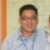Chew Jian Chieh

Everyone is taught in school the equation of a straight line:

Y = a + bX

Where a is the Y-intercept and b is the slope of the line. Using this equation and given any value of X, anyone can compute the corresponding Y.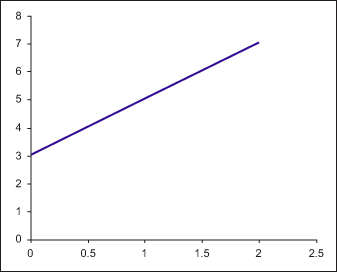Figure 1: Charting the Formula for a Straight Line

In Figure 1, Y = 3 + 2X. It is easy to see visually that a is 3. For the slope b, any two points on the line need to be chosen, say X1 = 1, Y1 = 5 and X1 = 2, Y1 = 7 and apply the following formula: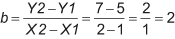### Method of Least Squares

Now suppose in real life the following data points are collected: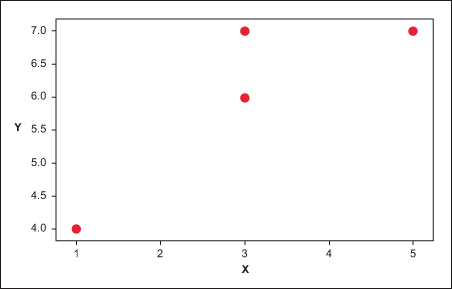Figure 2: Scatterplot of Y Versus X

How can one figure out the equation of the line that is drawn through the middle of these set of points? Statistically, the best fitted line is the one that minimizes the error between the points on the line (also called the fits or Y-hat) and the actually observed data points.

The easiest way to determine this line would be to calculate the sum of the differences between the fits (Y-hat) and the actual observed points (Y). But this method sometimes does not work because the positive and negative values may cancel each other out to obtain zero. A better method is to obtain the sum of the absolute difference. But this method does not stress the magnitude of the error.

However, if one squares the difference before they are added, two things are achieved:

1. It cancels the effect of having both positive and negative values
2. It magnifies (penalizes) the larger errors

Hence, one would choose the model with the least squares difference. But how can anyone tell if they have in fact found the best fitting line? Is there another line that will give an even lower least squares difference?

Statisticians have found that the line with the best fit has the following slope: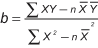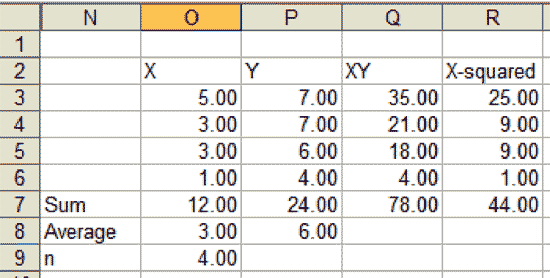To find a: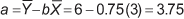Hence, Y-hat = 3.75 + 0.75X is the best fitted line.

### Standard Error of Estimate

Intuitively it is clear that a line is a better estimator of the data points when the points lie close to the line, than when they lie far away from the line. One needs a way to measure the scatter of the observed values around the regression line. This can be done via the standard error of the estimate: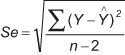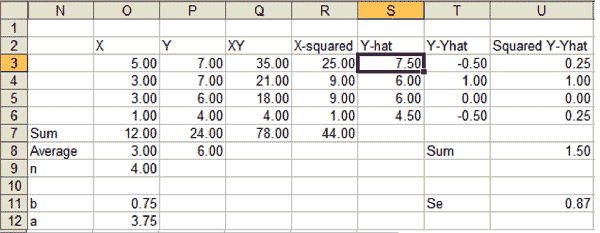The larger the Se, the larger the dispersion of the points around the regression line. In the Minitab output, this is given by the s symbol. Assuming the points are normally distributed around the regression line, one would expect 68 percent of the points within ± 1Se, 95.5 percent within ± 2Se and 99.7 percent within ± 3Se.

Handpicked Content:   Use Realistic Tolerancing and Poka-yoke for Process Control

### Coefficient of Determination and Coefficient of Correlation

One also can obtain the coefficient of determination, or R2 or R-Sq(uared). This is: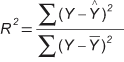And the coefficient of correlation or r is: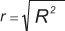R-squared provides the percentage of variation in Y that is explained by the regression line: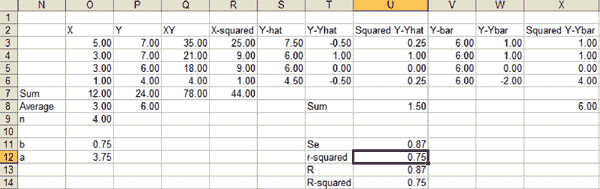Figure 3 shows the Minitab output of the same case showing the regression line, Se and R-Sq.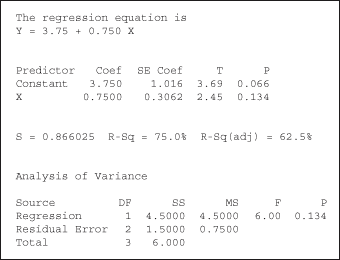Figure 3: Regression Analysis: Y Versus X

### Significance of the Model: The F Statistics

A 75 percent explained variation sounds pretty good. This model seems to be a representation of the data points. But is this really true? There are only have four data points – almost every line would look good if there were only a few data points? Therefore a much more important indicator of the validity of the model is – as always – the p-value.

The p-value in a simple linear regression is determined via the so-called F statistics: An F-value is calculated as the quotient of the variation that is caused and can be explained by the X in the model (in Minitab: mean of sum of squares for regression [MS regression]) divided by the variation that is caused by other variables which are not included in the regression, the error (in Minitab: mean of sum of squares for regression [MS residual error]). Logically, the more variation can be explained by the X and the less is unexplained the higher the F-value. In this case, F = 6. But is this already high enough to conclude that the variation explained by the X is significantly higher than the unexplained variation?

Handpicked Content:   Checking Simple Linear Regression Analysis Using 5S

In order to retrieve the p-value, one now uses the F-tables (easiest is to use is Excel’s FDIST function), for DF regression = 1 and DF error = 2. DF regression is 1 because there is only one X in this case. And since total DF as usual is n-1 (i.e., 3) DF error is 2 (= DF total – DF regression)

In this case, the p-value is 0.134. If alpha is set at 0.05, then one would have to reject this regression line as having a valid fit because p-value is greater than 0.05. This means that the model is not significant. The R-Sq value – though looking quite good –is of no value and should not be interpreted. Those who did this regression will need to collect more data, re-do the regression and then see whether the p-value is now significant before they interpret the R-Sq value.

##### Chew Jian Chieh

1.silerioj

The formula above for R-sq confuses me. I thought R-sq is the ratio of SSR over SST, which simply translates to the percent of the total variation due to the linear relationship between the dependent and independent variables.

2.Rafael Espinosa

For the R2 equation, shouldn’t you subtract the results of that equation from 1? The way you have it, it doesn’t work out…1.5 divided by 6 0.75, it equals 0.25.

3.Rafael Espinosa

Sorry, it should say:

1.5 divided by 6 does not equal 0.75, it equals 0.25.

4.Kicab

“if one squares the difference before they are added, two things are achieved:

It cancels the effect of having both positive and negative values
It magnifies (penalizes) the larger errors.”

You haven’t explained why it is necessary to penalize larger errors to find a line that “fits” or explains the data? You could penalize larger errors in many ways: multiply only large errors by a factor, use any even power, e.g., fourth and not just second power (square), use only errors that are greater than a certain percentages. In addition, by “penalizing” larger errors, those values will be substantially more influential in determining the line. The result is that a single point can make the least squares line be substantially misleading for most points.

The better explanation for squaring is that it provides additional and tractable statistical benefits: hypothesis testing and confidence intervals using unbiased estimates of the variances. However, this does not mean that the least squares line is the most useful one.

If your purpose is to find a line that contains either the averages or proportion of individual values within a predefined limit, other ways of determining that line are better. First, determine why you want to fit a line to data and then determine what method(s) will be better. You might choose the absolute deviation approach—or other methods found in the literature.

“Therefore a much more important indicator of the validity of the model is – as always – the p-value.” The p-value is probably the least important indicator of model validity.

Again, depending on the purpose, other criteria that evaluate the extent the purpose is met are better indicators. No one (I hope) has as a purpose to fitting a line to data that the p value be significant. Rather most often the purpose is to predict. Hence, the accuracy and frequency (probability) of correct predictions are better indicators for the prediction purpose.

Check Minitab for definition of influential points. You will see that one type is a point far from a fitted line in a vertical direction (Y). This influence is exaggerated using least squares. The other type is a point far from the others in a horizontal (X) direction. This will increase R-square and lead to mistakenly significant p-values.

5.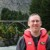Chris Seider

I always tell my folks to look at the p-value and R-sq. If the p-value is less than their typical 5%, the R-sq tells them what percent of the variation is explained but I always tell them to look at the RESIDUALS before getting excited. Make sure there’s no pattern or big outliers and a few other items.

Residual analysis is critical to successfully applying the tool.

6.LSSBB

For regression analysis, always use R-Sq (adj) instead of R-Sq to determine the goodness of fit, assuming the model has already passed the p-value test.